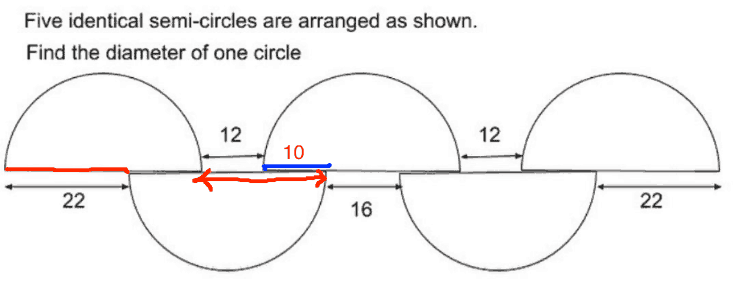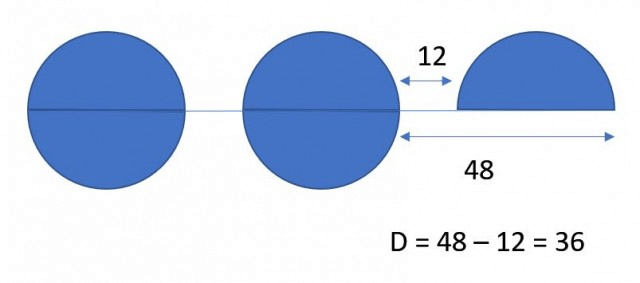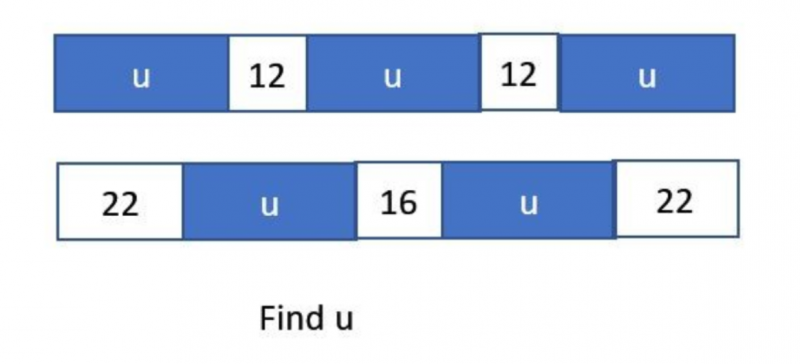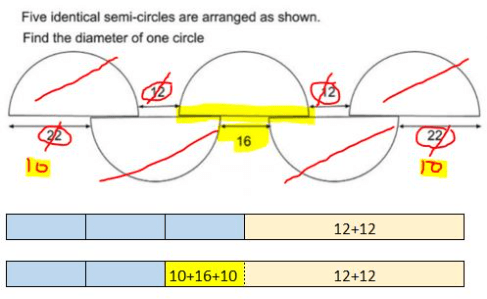# Answer

36

0 Replies 0 Likes

let diameter be D.

D=22 + X

D= 16+ 2Y

D=12+x+y

solve simultaneously

D=36

0 Replies 0 Likes

36

0 Replies 0 Likes

Method 1 :
Removing 2 semi-circles each from the top and bottom, diameter of one circle + 12 + 12 = 22 + 16 + 22. Diameter of one circle = (22 + 16 + 22) – (12 + 12) = 36

Method 2 :
Let the diameter of one circle be 1 unit.
1 unit + 12 + 1 unit + 12 + 1 unit = 3 units + 24
22 + 1 unit + 16 + 1 unit + 22 = 2 units + 60
3 units + 24 = 2 units + 60
1 unit = 36

Ans : 36.

0 Replies 0 LikesRed arrow length = 22

So blue length = 10

Diameter = 36

0 Replies 0 Likes1 Reply 0 LikesIf I draw this question in blocks as shown, I guess even P4 students can solve this ‘difficult’ PSLE questions. Numbers are the same, just different characters. NO semi circle, no diameter, just plain blocks and find u. So it’s not the kids don’t know how to solve, it’s they need to learn how to interpret the questions, and it’s only thru more exposure to these type of questions they will get better. So practice on more assessment papers. Brings questions here to your children to do.

0 Replies 2 LikesJust sharing my personal experience: My DD only managed to solve this half-way with algebra but with careless mistake. One of the tips I gave her before the night of PSLE Maths exams is to “Think Simply“. However, she didn’t apply it for this question. After the exams when she asked me, I cancelled out in the diagrams while explaining and she gave me the “blur” look. Then I tried another way and drew the bar model diagrams and said “you all drew these in P3” and all realisation came through. Nevertheless, I’m sticking to this principle for my next P6 kid in a few years’ time. Hope it still works by then.

0 Replies 1 Like

3D  + 24 = 2D + 60

3D – 2D = 60-24

1D = 36

0 Replies 0 Likes

The diameter of the semicircle is (22-12)cm x 2 + 16cm = 36cm. This is because when you look at the first two semicircles from the right, you’ll find there is a “line” where the two semicircles are “in contact” with each other. So, logically, if you take the diameter of the semi-circle and subtract the length of the length “in contact”, it will equal 22cm. It should work the same for the second semicircle from the right, but the length is only 12cm, a 10cm difference from 22cm. This means that the other part the second semicircle from the right has “in contact” with the third semicircle from the right is 10cm too. As this figure is symmetrical, you add the two 10cm lengths to the 16cm and voila, you get 36cm.

Hope this helped.

— Just another P6 student

0 Replies 2 Likes